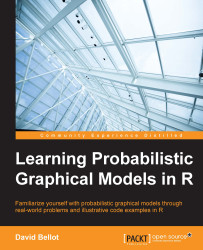•#### Learning Probabilistic Graphical Models in R#### Overview of this book

Probabilistic graphical models (PGM, also known as graphical models) are a marriage between probability theory and graph theory. Generally, PGMs use a graph-based representation. Two branches of graphical representations of distributions are commonly used, namely Bayesian networks and Markov networks. R has many packages to implement graphical models. We’ll start by showing you how to transform a classical statistical model into a modern PGM and then look at how to do exact inference in graphical models. Proceeding, we’ll introduce you to many modern R packages that will help you to perform inference on the models. We will then run a Bayesian linear regression and you’ll see the advantage of going probabilistic when you want to do prediction. Next, you’ll master using R packages and implementing its techniques. Finally, you’ll be presented with machine learning applications that have a direct impact in many fields. Here, we’ll cover clustering and the discovery of hidden information in big data, as well as two important methods, PCA and ICA, to reduce the size of big problems.
Learning Probabilistic Graphical Models in RCreditswww.PacktPub.comPrefaceFree Chapter
Probabilistic ReasoningExact InferenceLearning ParametersBayesian Modeling – Basic ModelsApproximate InferenceBayesian Modeling – Linear ModelsProbabilistic Mixture ModelsAppendixIndex## Summary

In the second chapter, we introduced the fundamentals of inference and we saw the most important algorithms for computing posterior distribution: variable elimination and the junction tree algorithm. We learned how to build a graphical model by considering causality, temporal relationships, and by identifying patterns between variables. We saw a fundamental feature of probabilistic graphical models, which is the combination of graphs to build more complex models. And we learned how to perform inference with a junction tree algorithm in R and saw that the same junction tree can be used for any type of query, on both marginal and joint distribution. In the last section we saw several real-life examples of PGM that can be used in many applications and are usually good candidates for exact inference.

In this chapter, we faced a problem when defining a new graphical model: the parameters are tedious to determine. In fact, even on small examples it's complicated. In the next chapter we...